4. Fundamental Periods of the World

L. G. Kreidik (translation from Russian T. S. Kortneva and G. P. Shpenkov)

4.1. A norm of the dominant ideal period

One of the mam channels connecting material ideal processes and states of the Universe is an ideal quantitative channel with its numerical affirmation-negation field, the field of material-ideal quantitative-qualitative dialectical numbers-measures.

In view of this, a question arises what exist basic periods in the World for connection of the Transient and Eternal, Material and Ideal, contents and form, quantity and quality, etc ?

This important question can be answered by history of Reason on the Earth, which is a component of the ideal aspect of the World. The answer can be obtained from analysis of measure units of different nations on the Earth, formed for millennia. These measures are objective as they were not affected by any theory and only in the two recent centuries they were distorted by establishment of the official national and international measures.

It will be assumed that the multiplicative discreteness root is ten. Indeed, decimal count was suggested to man by fingers and this suggestion can be attributed to Cosmos. Therefore, it should be expected that the norm of the dominant ideal period is equal to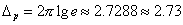(1.83)

within a decimal scale.

4.2. A spectrum of the Old Russian system of measures

As an object of our analysis we will choose Old Russian system of measures, the range of which should be described mainly by the formula,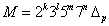, (1.84)

where numbers 2, 3, 5 and less frequently 7 are ordinary unties of count and k, I, m, n e Z The spectrum (1 84) is universal and inherent in ancient measures of many nations [1-18]

a) The measures of length.

The first natural units of simplest measures of length were fingers and their joints, palms, spans, feet, elbows and other parts of the human body. In the Old Russian metrology, a foot of about 27.3 cm and a finger of 2.73 cm, equal to a tenth of a foot, were constitutive measures

The measure equal to a foot is typical size of bricks, books, icons and architecture details in XI-XII centures. The mam derivative units of the foot was a sazhen in 4, 5, 6, 7, 8, 9 feet A sazhen = 5 feet = 3 elbows= 137 cm was the most widely used unit of length. Jakov's arquebus as cast in 1492 had length of a firearm barrel equal to 1.37 m. The distance between rowlocks in most of boats had often length 1.37 m. The measure in 1.37 was the typical length of oars in XIV centure. A sazhen=10 feet=2.73 m has known as a grand sazhen. It has been defined in the following way. A lace with the length of a grand sazhen has been folded in two and its midle point pressed by a hand to the shoulder, therewith, ends of the lace have to touch of a floor, because according to anthropology data a shoulder in average is on the height of 1.37 m.

b). Some measures of volume.

The main measure of volume in XVIII centure was the volume of a russian bucket, a vedro, equivalent to 13.7 litres. We will term this bucket as the canonical bucket. Along with a vedro have been existed vedroes multiple to the canonical vedro. Ten canonical vedroes with the volume 137 litres are related to the measure of mass 137 kg equal to an average value of a barrel of some countries.

c) The measures of mass and money.

Russian metrological spectrum of mass is closely related with wheat grain, which in Russia has called pirog. This word has been originated from the Old Russian name of wheat, pyro. According to historical and archaeological data, the Russian metrological spectrum of mass has been represented by the series:

1 pirog (pie) (a wheat corn) = 42.625 mg

1 polupochka (a half-bud) = 2 pirogs = 85.25 mg

1 pochka (a bud) = 4 pirogs = 0.1705 g

2 pochkas = 8 pirogs = 0.3411 g

4 pochkas = 16 pirogs = 0.6822 g (1.85)

8 pochkas = 32 pirogs = 1.3644 g

12 pochkas = 48 pirogs = 2.0466 g

16 pochkas = 64 pirogs = 2.7288 g

20 pochkas = 80 pirogs = 3.4110 g

24 pochkas = 96 pirogs = 4.0932 g

This series of units is described well by formula (1.84), simultaneously verifying it.

During formation of market this spectrum revealed itself in the medieval Russian currency system which lean on ingots of silver and gold or on grivna and coins of the East. Russian monetary manner had deallings generally with three groups of east coins with mass about 1.9-2.0, 2.73, and 4.0-4.2 g. All they, naturally, enters in the Russian metrological system.

The common Russian monetary count has been formed to the end of XV centure: 1 rouble = 100 kopecks = 200 money = 400 quarter-kopeck pieces = 800 half-quarter-kopeck pieces = 1600 pirogs = 3200 half-pirogs = 6400 qurter-pirogs = 68.22 g.

In XV-XVII centuries, money have simultaneously been used as units of mass based on 1 rouble mass equal to 68 22 g and its derivatives

Measures of volume and mass are closely interconnected and they select the values of the metrological spectrum. For example, the volume of gram of 137 litres corresponds to a measure ( an osmina) of 105 kg at typical specific volume of gram 1.31 l/kg. Such volume of water generates the measure ot mass 137 kg, i.e. ten basckets of water with the volume of 13.7 litres each.

Metrics similar to the Old Russian one have also be found in other nations. These ancient measures distinguish definitely the fundamental period, without which deep understanding of the Universal process is impossible. The dominant period pierces natural science, music, and art and it manifests itself by human perception of the environment.

4.3. Formation of fundamental gauges

a) The volumetric density and permeability.

Now, we will analyze the development ot fundamental gauges Folk gauges of mass and volume were developed on the basis of comparison ot masses and volumes of liquid and free-flowing substances Nature made ancient people compare the mass and volume of water with those of other materials In the epoch of initial land cultivation, water (wine, beer) and grain became main factors determining ancient natural measures.

Water gave rise to the formula which relates mass and volume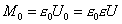, (1.86)

where M is the mass ot water equal to the mass of a substance, U0 is the volume of water, U is the volume of the substance,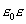is the volumetric density,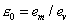is the unit of the volumetric density equal to the ratio of arbitrary unit of mass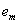to arbitrary unit of volume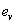,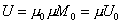will be called the relative volumetric density.

The inverse relation developed simultaneously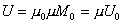, (1.87)

where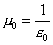is the unit of the mass density and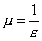is the mass density of substance, which will be also called the volumetric permeability of substance, because of interdependency both these characteristics.

Comparison of other materials with water in mass and volume gave rise to the relative volumetric density and permeability, independent on concrete choice of units of mass and volume. Consequently, most of nations on the Earth, comparing liquid and grain, created similar or rationally multiple measures. An alternative is hardly possible.

b) An influence of the golden section law.

Average anthropometric parameters of many nations played an important role in the appeared similarity of measures and many of them virtually coincided. Moreover, the law of the golden section affected much for the similarity of measures. Its essence is as follows. If an interval in the measures of possible random scatterings is selected and divided into sixteen equal intervals or metameasures, then nature most often chooses the right or left tenth metameasure. The left tenth metameasure will be called a subdominant and the right tenth metameasure, a dominant. When the interval is divided into eight metameasures, the subdominant is represented by the third metameasure and the dominant, by the fifth metameasure.

Choice of the dominant and subdominant occurs unconsciously. This phenomenon was noticed long ago and, in art, the similar selection of measures was called the golden section law, which has been formally explaining by the irrational ratio. In actual fact, the golden section law is based on the decimal base law and when the dominant selects the fundamental half-period, the length of the interval is often equal to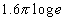. This can be found in formats of books. For example, the height of covers of most Russian books is equal to a great span with the canonical measure: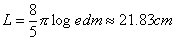. (1.88)

The fifth metameasure of 13.7 cm is most often used for the title of a book.

We will consider dialectics of the choice of the unit of one millimeter in terms of the law of the golden section. Acuteness of vision, corresponding to the least distance between two points, that can be discerned by a human eye, is equal approximately to one angular minute. At a distance of the best vision, the segment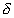corresponds to this minute It selects the golden section in one millimeter by the five fundamental periods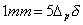. (1.89)

Thus, a millimeter is a magic unit.

Comparison of the modern data concerning the relative volumetric densities of grain crop with ancient measures shows that the average relative volumetric permeabilities of gram were approximately equal to a half of the fundamental period: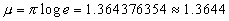. (1.90)

Accordingly, the relative volumetric density was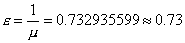. (1.91)

Hence, we obtain the relation between the mass of gram and its volume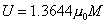,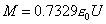. (1.92)

If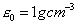is assumed, then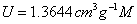,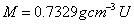. (1 92a)

4.4. British ineasures

British measures that had a great effect on official national measures of many countries will be analyzed in terms of the above relations and the fundamental period A yard, a basic unit of length of English-speaking peoples was established by Henry I (1101) to be equal to the distance from the tip of his nose to the finger-ends of his stretched arm At present, a yard is equal to 91.44 cm Here are the main measures of length of this system

1 fathom = 2 yards 1 hand = 4 inches

1 yard = 3 feet 1 palm = 36 lines

1 cubit = 18 inches 1 nail = 27 lines

1 foot =12 inches 1 inch =12 lines

1 span = 9 inches 1 line = 6 points

An inch, equal to the middle width of the thumb, was established by Edward II in 1324 and defined as the length of three barleycorns, from the middle part of an ear, lined up by their ends:

1 inch = 3 barleycorns

1 barleycorn = 4 lines.

In Russia, Peter's I edict equalled a Russian sazhen (fathom) to seven English feet. This edict resulted in some changes in the Russian system of measures, which became:

1 sazhen (fathom)=3 arshine=7 feet

1 arshine = 4 quarters = 16 vershoks

1 foot = 12 inches = 120 lines = 1200 points.

A Russian merchant pound was revised to be the mass 25.019 cubic inches of water at its highest density and division of the pound into 32 lots was introduced. Apart from the trade pound in use was an apothecaries' (pharmaceutical) or a Nurenberg pound equal to a Roman libra of 84 zolotniks or 409.51241 g. In ancient Rome an ounce was equal to 27.288 g and the apothecaries' pound was equal to 15 ounces but it does not mean that a Nurenberg pound by origin is ancient Roman.

The payment ingots with the mass of about 0.188 kg were used in the 12-15th centuries in Velikiy Novgorod . These ingots were related to the unit of volume 0.188 kg, which corresponds on the average to the mass of grain 0.137 kg with the relative volumetric density 0.7329. At the same volumetric density, the ingots by mass of about 200 g defined the volume of grain 0.137 l, which, in turn, defined mass 274 g. Thus, the ingots were closely connected with the fundamental period and a ruble by 68.44 g.

As was mentioned above, a British foot was appeared in Russia on base of division of a Russian measuring plank: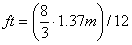.

Thus, an ideal measure of a British pound is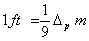.

This measure describes most accurately the ancient system of British measures of length with an ancient natural foot of 2.73 dm and a Flemish elbow of 2.5 ft, equal to 0.137 m. Famous British metrologist Chaney claimed that British measures of weight and linear measures, like measures of the other European countries, had originated, apparently, from the East, coming to Europe through Greece and Rome, and their origin was almost prehistoric . Another British metrologist Chisholm wrote that it was thought that Egyptian units of weight and linear units had come to Asia, India and later to Greece and, with some modification, spread in Italy, where they were been adopted by Romans and later by all the other European nations . These statements are not indisputable. It is most likely that the second-kind law of the fundamental period and the decimal base everywhere play the decisive role.

With this in view, we will analyze some British measures, bearing in mind that the volumetric density of cereals in Great Britain was in the range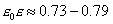kg/l.

For the volumetric density 0.75 kg/I, a bushel of free-flowing materials defines a unit in one bushel of mass as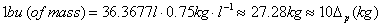,

a tenth part of which is equal to the fundamental measure that lay in the basis of the Oriental measures. Through liquids (water, wine, beer), the bushel of mass, in turn, formed an equal by value the bushel of capacity 27.28 l. Three pecks were virtually equal to this bushel. Further, like pounds of capacity 0.373242 l with a volumetric density defined by (1.92), British apothecaries' and monetary pounds gave rise to pounds with the mass 0.273 kg. One hundred of these pounds composed a bushel of mass. Five bushels of mass generated the barrel 136.4 kg. A Japanese koku of grain of 136.88 kg, a British tierce of meat of 137.89 kg, an Australian bale of wool of 136 kg, and numerous barrels of petroleum products are related to the same spectrum of measures.

Other examples, in Iran a barrel is equal to 136.4 kg, in Brazil, to 136.7 kg, on the Bahrain Islands, to 136.3 kg, in Kuwait, to 137.8 kg, etc. In terms of the USA wine barrel 119.24 / and the British barrel of the bulky materials (grain) 163.65467 l, the average relative permeability of cereals in the British metrological system will be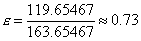,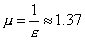.

This value is very close to the canonical measures (1.92), therefore, during the very long history of the material and spiritual British culture, measures similar to the most ancient Oriental units must have developed and they did. The East does not seem to play a decisive role here, otherwise the Ancient Roman ounce, virtually equal to the fundamental period of 2.7288 dg, should had laid at the basis of British measures.

4.5. Measures of ancient Babylon, Egypt, Rome, Greece and Chinese

In ancient Babylon minas of mass, proportional to Roman ounces were widely spread:

1 mina = 15 ounces = 409.3129 g

1 mina = 18 ounces = 491.1755 g

1 mina = 20 ounces = 545.7505 g.

In ancient Egypt a kedet was the main unit of mass:

1 kedet = 1/3 ounce = 9.09584 g ~ 9.096 g

In ancient Rome a libra of mass was equal to twelve ounces:

1 libra = 12 ounces = 327.4503 g.

Pounds were also in use there:

1 pound = 10 ounces = 272.8753 g,

1 pound = 30 ounces = 818.6258 g,

1 pound = 35 ounces = 955.0634 g,

1 pound = 60 ounces = 1637.2516 g.

In ancient Greece a metret (a unit of capacity) was equal to 1000 ounces or capacity:

1 metret = 27.2878 l,

1 metret =100 kotylas (cups).

Kotylas gave rise to an amphora:

1 amphora = 72 kotylas = 16 pecks = 36 mugs = 19.647 l.

An amphora of mass was a unit of monetary weight:

1 talent = 60 minas = 19.647 kg,

1 mina = 100 drams = 600 obols = 327.4503 g.

In ancient Attic a talent of a larger mass was in use:

1 talent = 80 minas = 26.196 kg.

In the Middle Ages a grivna with mass of 233.769 g was in use. As a unit of capacity, it determined the golden section of the fundamental pound 272.88 g: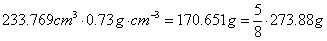.

Now, we will go to the other extremity of the Eurasian continent and dwell upon the Chinese metrology. Chinese measures of length are closely connected with rice, the first information about cultivation of which had appeared 2800 years before AD . The transverse dimension of a rice corn [22, 23] varies in the range d = 1.2-3.5 mm. The subdominant and dominant of the range are 2.06 mm and 2.64 mm, respectively. It is possible that ten subdominants gave rise to an inch 2.06 cm. Chinese feet must have originated from this inch:

1 foot = 12 inches = 24.72 cm,

1 foot = 16 inches = 32.96 cm,

1 foot = 18 inches = 37.08 cm.

The above calculations are consistent with ancient Chinese feet. In particular, a long Chinese foot is equal to 37.5 cm. A foot of 16 inches is close to a building foot of 32.28 cm. A foot of 12 dominant inches (31.68 cm) coincides actually with a landmark or engineering foot of 31.97 cm. An average Chinese foot is about 32.8 cm. If it is divided into 16, we will obtain one of the ancient Chinese inches; if the foot is divided into 12, we will have an inch of 2.73 cm close to the fundamental measure.

A Chinese lan of mass 37.35 g corresponds to a lan of capacity 0.03735 l, defining the fundamental period of mass 27.3 g with the relative volumetric density 0.73. The average relative volumetric density of Chinese rice equal to the ratio of a dan of liquid capacity to a dan of grain capacity :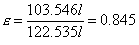,

gave rise to another series of measures based on rice. We can see that in China which is located so far from Great Britain, dialectics of measures is similar to that of European and Oriental measures.

Theoretical Dialectical Journal: Physics-Mathematics-Logic-Philosophy, N.1, site http://www.tedial.narod.ru/